# 【实战问题】-- 缓存穿透之布隆过滤器（1）## 为什么需要布隆过滤器？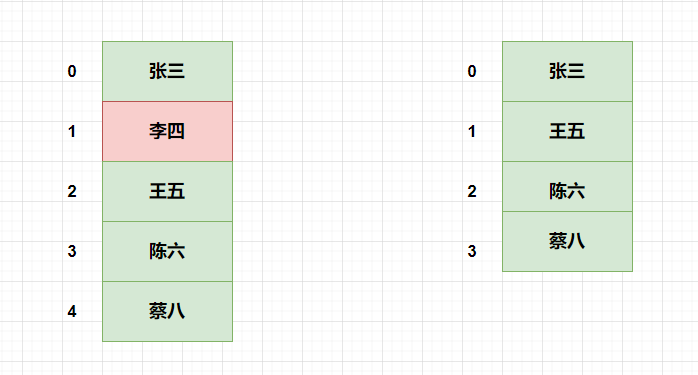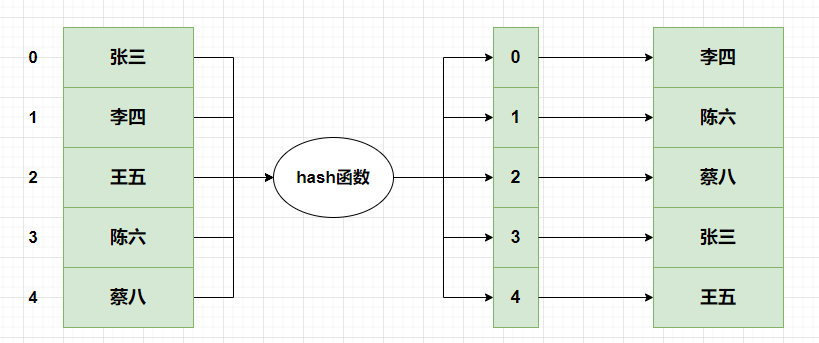• 只有一个 hash 函数，其实两个元素 hash 到一块，也就是产生 hash 冲突的可能性，还是比较高的。虽然可以用拉链法（后面跟着一个链表）的方式解决，但是操作时间复杂度可能有所升高。

• 存储的时候，我们需要把元素引用给存储进去，要是上亿的数据，我们要将上亿的数据存储到一个 hash 表里面，不太建议这样操作。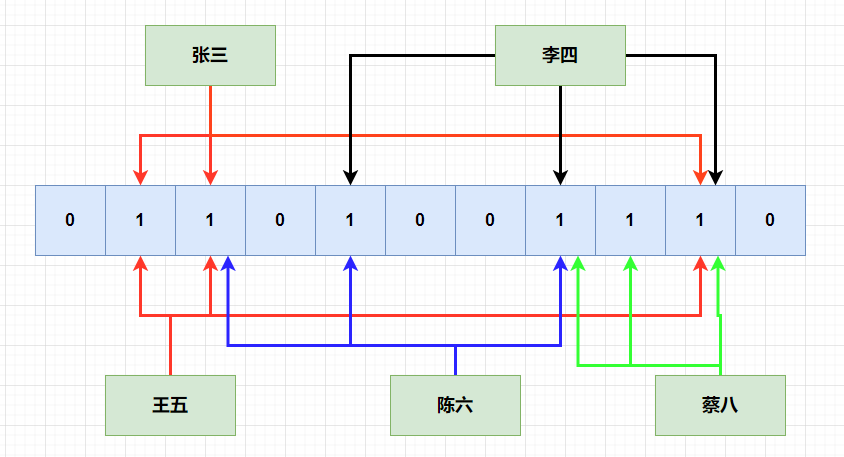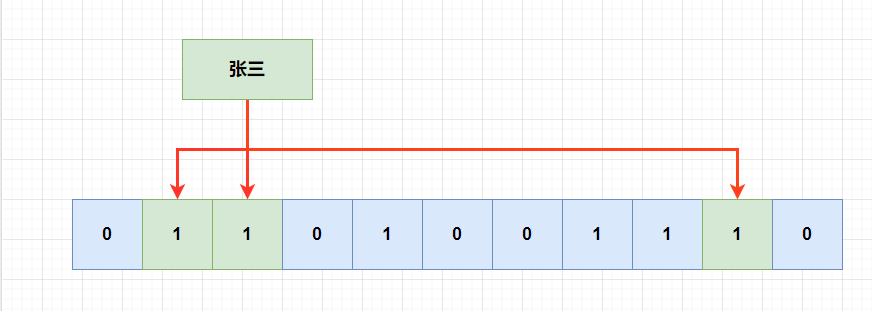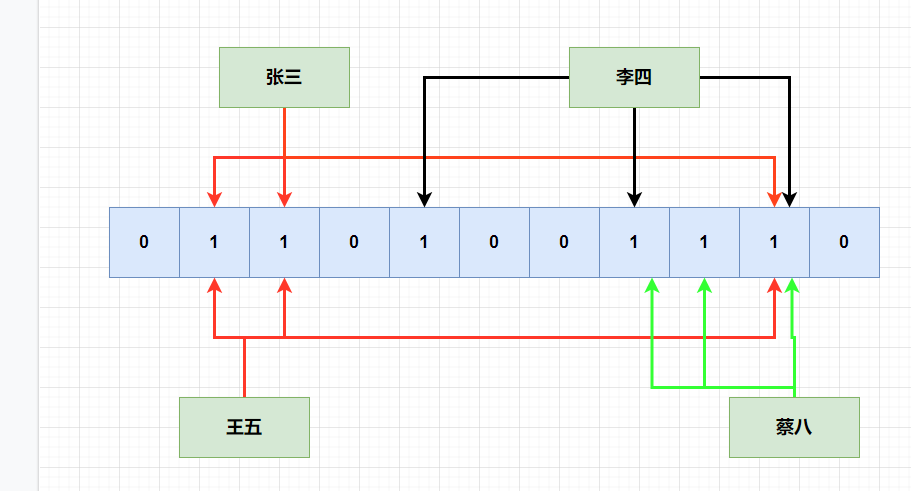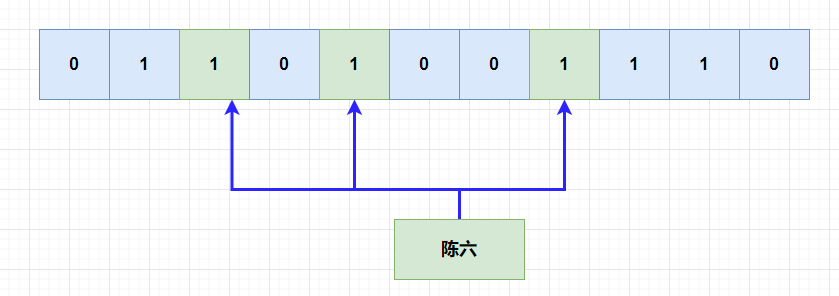## 具体步骤

• 1. 使用多个 hash 函数对元素 item 进行 hash 运算，得到多个 hash 值。

• 2. 每一个 hash 值对 bit 位数组取模，得到位数组中的位置索引 index。

• 3. 如果 index 的位置不为 1，那么就将该位置为 1。

• 1. 使用多个 hash 函数对元素 item 进行 hash 运算，得到多个 hash 值。

• 2. 每一个 hash 值对 bit 位数组取模，得到位数组中的位置索引 index。

• 3. 如果 index 所处的位置都为 1，说明元素可能已经存在了。

## 误判率推导

$$1-\frac{1}{m}$$

k 个 hash 函数都 hash 之后，该位还是没有被 hash 到 1 的概率是：

$$(1-\frac{1}{m})^k$$

$$(1-\frac{1}{m})^{kn}$$

$$1-(1-\frac{1}{m})^{kn}$$

$${(1-(1-\frac{1}{m})^{kn})}^{k}\approx {(1-e^{-kn/m})}^k$$

$$m=-(\frac{nInP}{(In2)^2})$$

## 布隆过滤器的作用

【刷题笔记】

Github 仓库地址：https://github.com/Damaer/codeSolution

【作者简介】## 评论